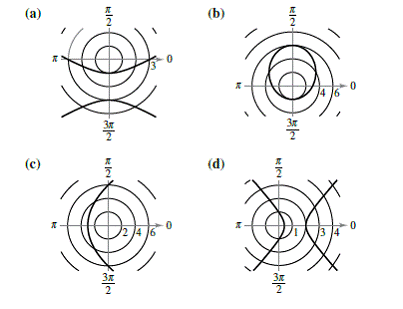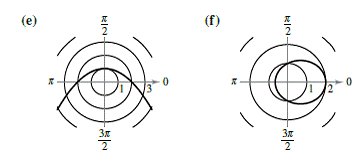Chapter 10.6, Problem 7E

Chapter
Section
Textbook Problem

Matching In Exercises 7-12, match the polar equation with its correct graph. [The graphs are labeled (a), (b), (c), (d>, (e), and f).]r = 6 1 − cos θ

To determine

Match the given polar equation with its correct graph from the options given.

Explanation

Given: Given options for graphs are labelled as (a), (b), (c), (d), (e) and (f) are shown below:

Calculation: The given equation is r=61cosθ

Here e=1, so the graph of the equation is a parabola. The form of the given equation concludes that the directrix is vertical and is at the left of the pole.

Since d=6, the directrix line is x=6

Still sussing out bartleby?

Check out a sample textbook solution.

See a sample solution

The Solution to Your Study Problems

Bartleby provides explanations to thousands of textbook problems written by our experts, many with advanced degrees!

Get Started

23(4x2x21)+3(33x1)

Applied Calculus for the Managerial, Life, and Social Sciences: A Brief Approach

Use definitions and properties of exponents to complete the following.

Mathematical Applications for the Management, Life, and Social Sciences

Write in cylindrical coordinates, where E is the solid at the right.

Study Guide for Stewart's Multivariable Calculus, 8th

The polar form for the graph at the right is:

Study Guide for Stewart's Single Variable Calculus: Early Transcendentals, 8th